### RELATIVITY

#### (With Math Derivations for Nether and Einsteinian Relativity)

##### Back to Time - Main Menu

[It is recommended that the portions of this website called Time and The Vortex be studied before studying the following.]

Insofar as the equations go, Einstein's theory of Special Relativity is correct.   However, Einstein used the Lorentz equations for his theory, so it is probably more correct to say that the Lorentz' equations, called the Lorentz transforms, are correct.   The point is that relativity works provided one understands that everything is relative to the nether.

Before going any further, please review the part of electron theory called The Mass/Energy Equivalency Equation which explains the vortex that is the electron.

If the velocity of a spaceship relative to the nether approaches the speed of light, then the subjective time (that of one who is on board the spaceship) approaches zero, meaning that the passage of subjective time is slowed so that any increment of time, such as one second, is lengthened.   According to Nether Theory (Einstein had a different interpretation), this causes the subjective effect of foreshortening the depth of space around the spaceship which means that the dimension of any distance or length that is in a straight line away from the spaceship appears to be foreshortened.   The crucial part of the Einsteinian relativistic equations and the nether equations is credited to Lorentz and is based upon the Pythagorean theorem.   It is called the Lorentz factor and is represented here as Y.
Previously in Book Five:   Y = 1 / [1 - (v2/c2)]1/2

In Time Dilation (TD) [part of a previous chapter in Book Five] it was shown how the Pythagorean theorem could be used to derive the Lorentz factor, and how the Lorentz factor relates to velocity and time.   A similar derivation can be used to show how the Lorentz factor relates to velocity and mass.

Before we begin to this derivation, you should understand that everything in this universe is composed of nether.   When we move an object made of vortices of nether, we use forces which operate no faster that the speed of light.   "c" is the highest velocity with which these forces can operate.   Lightspeed for a vorticle cannot be actually attained because greater and greater energy must be used to move it.   This is because our energy operates at the no more than the same speed that we wish to impart to the vorticle.   Therefore, the amount of energy needed to move something so small as an electron to the speed of light is infinite.   Think of it as analogous to blowing on a sailboat to make it go faster than sound.

Because lightspeed is the greatest velocity that can be approached and is actually a theoretical velocity which cannot be achieved, the amount of energy used may be found by the equation ET = YE0, where ET is its total energy and E0 is its rest energy (mc2) relative to the nether.   The way energy should relate to arrive at the foregoing can be shown visually by using a right triangle in which one side is E0, the other side is EK (kinetic energy), and the hypotenuse is ET.
Thus, E02 + EK2 = ET2.

When an object such as the electron is at rest (perfectly still) relative to the nether, there is no EK.   This means that there is no side to be shown for kinetic energy, so we show the "triangle" as only a vertical line which is supposed to be E0 lying on top of ET.   Next, we anchor the bottom ends of E0 and ET so that ET may rotate counterclockwise.   This vertical line is two vectors (one on top of the other) which illustrate how E0 and ET are equal when the the electron is at rest relative to the nether. [In reality, the electron is never completely at rest relative the nether.]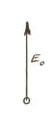If the electron now begins to move at relativistic speed (very high speed), the inflow pattern changes measurably due to nether pressure in front increasing and the fact that less can come into the vortex from the "sides".   We may represent this with another vector attached by its tail end to the top of the vector we have called E0.   This new addition is the vector we will label EK for "kinetic energy" and we draw it horizontally to the left.   This vector causes the ET to rotate counterclockwise because motion creates kinetic energy which adds vectorially to the rest energy of the electron.   The kinetic energy is added at ninety degrees because the rest motion of the incoming nether was largely from the sides and this shifts the average incoming nether motion so that it comes in more from the "front" or leading portion of the moving electron.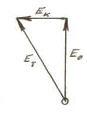As the speed of the electron relative the nether increases, the EK vector lengthens to the left and the ET vector rotates farther counterclockwise.   As ET rotates, it grows longer.   This is a geometric way of showing that total energy is increasing due to the increase in kinetic energy.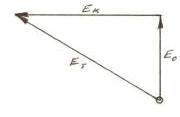As EK continues to grow longer, causing ET to continue rotate counterclockwise, we see that ET grows longer.   When ET is almost horizontal, both EK and ET are miles long and way off the paper.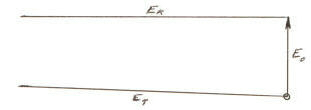If ET were to rotate to the horizontal, EK and ET would both be at right angles to E0 and extend to infinity without connecting, showing that both kinetic and total energy would be infinite.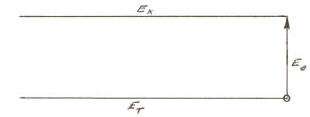The energy required to move an object to a very high velocity relative to the nether is what we have been considering.   We live in a universe where energy is conserved.   This means that the energy imparted to an object is kept by it.   So if the object were suddenly stopped, it would give up the kinetic energy it had acquired.

Momentum works the same way in that total momentum equals rest momentum times Y, indicating that energy and momentum equations have a common input.   Without this explanation becoming too lengthy, there is a reason why these things occur.   We have two types of input for energy and momentum:   mass and velocity.   The velocities used in the energy equations are squared.   The velocities used in the momentum equations are not squared, and this is contrary to what would be the case for a common input.   The only input that is common and thus can vary correctly in these equations is mass.   With this is mind, we can construct another right triangle.   [Remember that mass is merely a form of energy so the energy triangle above with its rotating vector is also the mass triangle.]

In the remainder of this explanation, "at rest" and "velocity" mean relative to the nether.

You may recall from book three of this series, that at the "edge" or boundary of the electron center the nether moves in at a velocity of "c".   The tangential velocity here is also "c".   Their resultant is "(21/2)c".   When the electron is at rest, it has no kinetic energy.   Its rest energy is found as the kinetic energy of the resultant of the Mass moving at the boundary,

E0 = (1/2)m(21/2c)2.

This is the same as the "mc2" of Einstein's relativity.
[E0 = (1/2)m(21/2c)2 = (1/2)m(2c2) = mc2]

m = M/T0, and T0 is defined as a time interval (such as one second) as experienced by an observer who is at rest relative to the nether.   We are going to be using three kinds of mass: rest mass, kinetic mass, and total mass, respectively "m0", "mK", and "mT".   "m0" is the amount of Mass which flows into the fourth-dimensional hole in one second when the electron is at rest.   "mK" is the increase in less restricted Mass flow due to the movement of the electron shifting more flow to the "front."   "mT" is the vectorial sum (the hypotenuse) of "m0" and "mK".   It is equal to m0 when the electron is at rest, and changes when "mK" is added as the electron moves.   When the electron is at rest or moving slowly relative to the nether, the following is true.

1.     Nether moves into the electron along an area that is an approximate hemisphere whose boundary is where nether "velocity" is equal to lightspeed.   Velocity is in quotes because the nether outside the electron is accelerating at all points and true velocity requires constant rate of motion for at least a very short time.   The area of the hemisphere is "(2pi)r2".

2.     The nether is accelerating at all points as it moves toward the electron center, from an infinite distance from the center.   This acceleration requires energy for it to continue, and the energy is furnished by the nether pressure versus the vacuum at the electron center.   Were this differential pressure to cease, any nether motion inward would also cease because acceleration requires energy.

3.     The tangential "velocity" vector at the boundary of the hemisphere equals "c".   The resultant of the inward and tangential "velocity" vectors is "(21/2)c".

4.     At the electron center, the incoming nether from any direction within our 3D universe must turn 90 degrees to move into the fourth dimension.   This is what causes the electron vortex to form.   So all of the incoming nether is turning 90 degrees.

5.     Its rate of flow into the electron is expressed as

"M/T0" which is "DV/T0".

"D" is density and "V" is volume.

"A" is area and "d" is distance moving inward.

d = vT0.

"v" is velocity which, in this instance is "c".

Since density does not change appreciably for nether in this instance except possibly for non-related causes, we can let it be equal to one and eliminate it from the equation.

So m0 = M/T0 = V/T0 = Ad/T0 = Av = (2pi)r2c.

mK = 0.

So mT = m0 = (2pi)r2c.

As the electron begins to move, any inward velocity vector of the nether cannot remain at "c" from any direction perpendicular to the electron movement, so the average of the inward flow shifts direction toward the "front" of the electron (the part foremost as the electron moves).   If lightspeed could be attained, there would be no nether flow from the sides as it would all come from the front.   The inward velocity vector would be from the front, the tangential velocity vector would remain as it was, and the resultant velocity would have shifted to become 45 degrees from the direction of electron motion.   At lightspeed the following would be true.

1.     The area at the center of the electron where nether enters would be a disc with an area of (pi)r2. The disc area would have moved in front and out to infinity, causing the inflowing nether to be like a tunnel with a cross-section equal to the area of the disc.   The tunnel would be infinite in length, causing the Mass of nether coming in from the front to be infinite.   Thus, the electron would be shaped like a disc with an infinitely long cylinder in the front of it.   If we define the cylinder as part of the electron, it is mass [which is Mass divided by an infinitely long second (mass equals Mass per time - and the passage of time has slowed to zero)].

2.     The electron hole, moving at "c", would still have the usual pressure differential to cause the boundary "velocity" vector to be "c", but the pressure from the motion would cause an additional differential pressure of "c", so their total would be "2c".   The velocity of nether inside the tunnel would be "c" relative to the nether around the tunnel and "2c" relative to the fourth-dimensional hole.   "Av" which is "m0" would still be "(2pi)r2c", but there would be an infinite volume of nether moving at "c" relative to the adjacent nether.   This infinite length (and infinite volume of Mass) in motion is "mK".   This means that "mT" would also be infinite.

3. The tangential velocity would still be c because the nether flow from the front must turn 90 degrees to enter the fourth dimension.   The resultant velocity relative to the nether outside the electron cannot exceed "(21/2)c", so there can no longer be any nether movement into the electron from any direction perpendicular to its direction of motion.

If the electron were suddenly stopped, the incredible volume of Mass flowing from the front would stop.   Unlike the flow when the electron is at rest, this flow is not restricted by the funnel effect and it carries infinite momentum and energy.   So the kinetic energy of this Mass would be converted back to the energy that was used to increase the electron velocity.

Electron Approaching the Speed of Light

The mathematical version of what is happening as an electron or any other form of matter approaches lightspeed can be shown with a right triangle with sides which are "m0" and "mK", and a hypotenuse which is "mT".   This illustration is essentially the same as the one for energy except that the labels for the vectors have changed.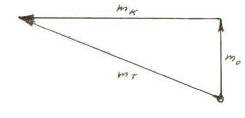m02 + mK2 = mT2     From the Pythagorean theorem.

mT2 - mK2 = m02     From rearranging.

(mT2/mT2) - (mK2/mT2) = m02/mT2     Dividing both side by "mT2.

[1 - (mK2/mT2)] = m02/mT2

mT2[1 - (mK2/mT2)] = m02     Multiplying by both sides by "mT2".

mT2 = m02/[1 - (mK2/mT2)]     Dividing both sides by the expression in brackets.

We know that the ratio mK/mT = v/c, so by substitution:

mT = m0/[1 - (v/c)2]1/2

mT = Ym0     This is the formula for total mass.

If we multiply both sides of the equation by "c":     mTc = Ym0c.

And if we multiply both sides of the equation by "c2":     mTc2 = Ym0c2.

Now we have the equations for mass, momentum, and energy for an object moving at a high velocity relative to the nether.   Einstein's equations are the same as the equations for nether theory, but in nether theory we are speaking of relativity as all being relative to the nether and Einstein was thinking in terms of one reference frame being relative to another.   Of course, at lower velocities relative to the nether, the effects are not very apparent.

The mass increase due to velocity can be found be subtracting the rest mass from the total mass.   The momentum increase due to velocity can be found by subtracting the rest momentum from the total momentum.   And the kinetic energy can be found by subtracting the rest energy from the total energy.